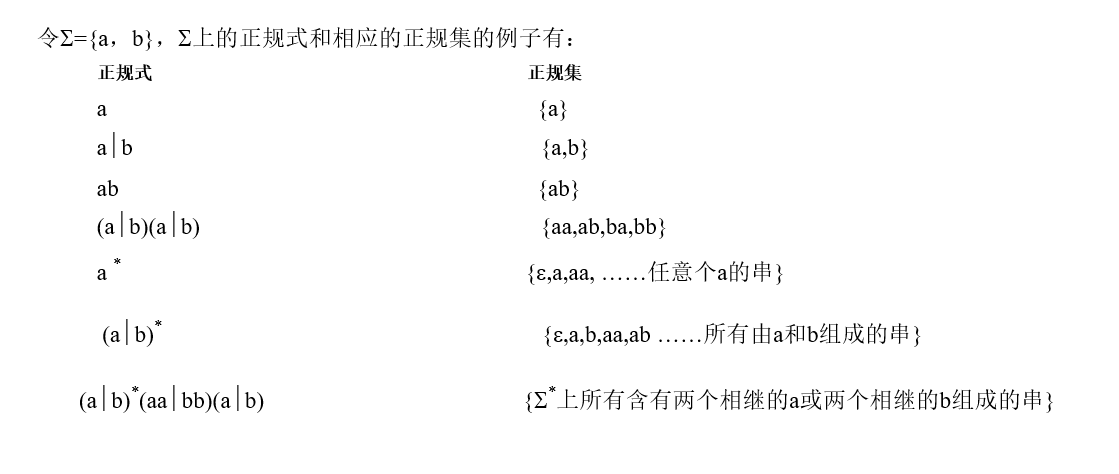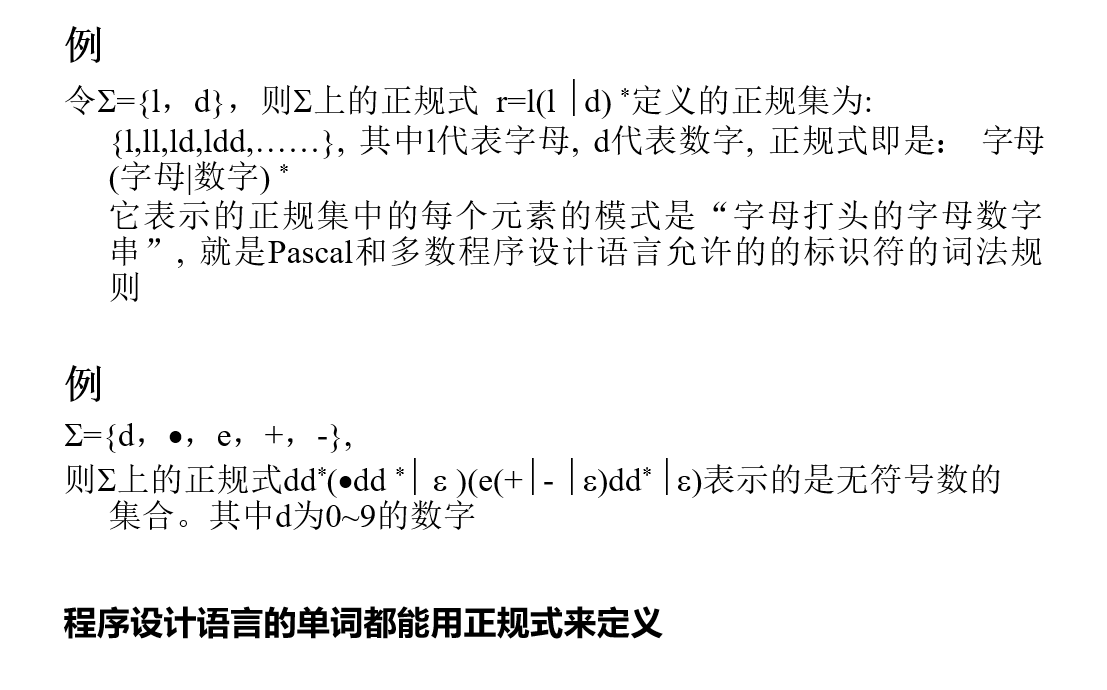# 编译原理 词法分析

Posted on 2020-10-20  111 Views

## 一、正规文法和正规式

### 1、文法与自动机的关系• 0型文法（短语结构文法）：其能力相当于图灵机，可以表征任何递归可枚举集，而且任何0型语言都是递归可枚举的。
• 1型文法（上下文有关文法CSG）：产生式的形式为α_1Aα_2→α_1βα_2，即只有A 出现在 α_1α_2 的上下文中时，才允许 β 取代 A 。其识别系统是线性有界自动机。
• 2型文法（上下文无关文法CFG）：产生式的形式为 A→ββ取代A时与A的上下文无关。其识别系统是不确定的下推自动机。
• 3型文法（正规文法RG）：产生的语言是有穷自动机（FA）所接受的集合。

### 2、正规文法与正规式

- 正规文法（3型文法）
- 正规式（正则表达式）

1. Φ 和 ε 都是 ∑ 上的正规式，它们所表示的正规集分别为 {ε} 和 {} ；
2. 任何 a∈∑，a 是 ∑ 上的一个正规式，它所表示的正规集为 {a} ；
3. 假定e1 和 e2 都是 ∑ 上的正规式，它们所表示的正规集分别为 L(e1) 和 L(e2) ，那么，(e1) , e1| e2, e1•e2, e * 也都是正规式,它们所表示的正规集分别为
L(e1) , L(e1)∪L(e2), L(e1)L(e2) 和 (L(e1)) *
4. 仅由有限次使用上述三步骤而定义的表达式才是 ∑ 上的正规式，仅由这些正规式所表示的集合才是 ∑ 上的正规集。

- “ | ” 读为“或”（也有使用“ + ”代替“ | ” 的）；
- “ ” 读为 “连接 ”；
- “ * ”读为“闭包”（即任意有限次的自重复连接。### 3、正规式等价

{e_1= b(ab)^* , e_2 = (ba)^*b}

e_1= (a|b)^*
, e2=(a^*|b^*)^*

1. “或”服从交换律：r|s=s|r
2. “或”的可结合律：r|(s|t)=(r|s)|t
3. “连接”的可结合律：(rs)t=r(st)
4. 分配律：r(s|t)=rs|rt(s|t)r=sr|tr
5. 零一律(ε是“连接”的恒等元素)： εr=rrε=r
6. “或”的抽取律: r|r=rr^*=ε|r|rr|…

### 4、正规式到正规文法### 5、正规文法到正规式

G=(V_N,V_T,P,S) ,存在一个∑ =V_T 上的正规式r : L(r)=L(G)

• $A→xB, B→y ≈ A=xy$
• $A→xA|y ≈ A=x→y$
• $A→x|y ≈ A=x→y$## 二、自动机

• 确定的有穷自动机(Deterministic Finite Automata) ：DFA

• 不确定的有穷自动机(Nondeterministic Finite Automata) ：NFA

### 1、DFA : 确定的有穷自动机

DFA定义

1. K 是一个有穷集，它的每个元素称为一个状态；
2. Σ 是一个有穷字母表，它的每个元素称为一个输入符号，所以也称Σ为输入符号表；
3. f 是转换函数，是在 K×Σ→K 上的映射，即，如 f（ki，a）=kj ，（ki∈K，kj∈K）就意味着，当前状态为ki，输入符为a时，将转换为下一个状态 kj ，我们把 kj 称作 ki 的一个后继状态；
4. S∈K是唯一的一个初态；
5. Z⊂K是一个终态集，终态也称可接受状态或结束状态。* 上的符号串 t 在DFA M上运行：

• 一个输入符号串t，（将它表示成 Tt的形式，其中T∈∑，t1∈ ∑ * ）在DFA M=（K，Σ，f，S，Z）上运行的定义为：

f（Q， Tt1）=f（f（Q，T），t1），其中Q∈K 扩充转换函数 f 为 K×Σ * → K上的映射，且： f（ki，ε）= ki

* 上的符号串 t 被 DFA M接受：

• M=（K，Σ，f，S，Z）

若t∈ ∑ * ，f（S，t）=P，其中S为M的开始状态，P ∈ Z，Z为终态集，则称t为DFA M所接受（识别）。DFA M所能接受的符号串的全体记为L(M)

∑ 上一个符号串集 V⊂∑ * 是正规的，当且仅当存在一个 ∑ 上的确定有穷自动机M，使得 V=L(M)

DFA的确定性表现在转换函数f:K×Σ→K是一个单值函数，也就是说，对任何状态k∈K，和输入符号a∈Σ，f(k,a)唯一地确定了下一个状态

### 2、NFA : 不确定的有穷自动机

NFA M=(K，∑，f，S，Z)，其中:

1. K为状态的有穷非空集
2. ∑为有穷输入字母表
3. f为 K×∑* 到 K 的子集（2K）的一种映射
4. S⊆K 是初始状态集
5. Z⊆K 为终止状态集f为 K×∑* 到 K 的子集（2K）的一种映射* 上的符号串 t 在NFA M上运行:

• 一个输入符号串t，（我们将它表示成Tt1的形式，其中T∈∑，t1∈ ∑*）在NFA M上运行的定义为：
f（Q，Tt1）=f（f（Q，T），t1） 其中Q∈K

*上的符号串t被NFA M接受：

• 若t ∈ ∑*，f(S0，t)=P，其中S0 ∈S，P ∈ Z，则称
t为NFA M所接受（识别）
• 也可以这样理解：

对于 Σ * 中的任何一个串 t，若存在一条从某一初态结到某一终态结的道路，且这条道路上所有弧的标记字依序连接成的串(不理采那些标记为 ε的弧)等于 t ，则称 t 可为NFA M所识别(读出或接受)。

若M的某些结既是初态结又是终态结，或者存在一条从某个初态结到某个终态结的道路，其上所有弧的标记均为 ε，那么空字可为 M 所接受。

NFA M所能接受的符号串的全体记为L(M)

∑ 上一个符号串集V ⊂ ∑ * 是正规的，当且仅当存在一个 ∑ 上的不确定的有穷自动机M，使得 V=L(M)• DFA是NFA的特例。对每个NFA N一定
存在一个DFA Ｍ, 使得 L(M)=L(N)；
• 对每个NFA N存在着与之等价的DFA M
• 有一种算法,可以将NFA转换成接受同样语言的DFA.这种算法称为子集法
• 与某一NFA等价的DFA不唯一

NFA到相应的DFA的构造的基本思路是：
- DFA的每一个状态对应NFA的一组状态

DFA使用它的状态去记录在NFA读入一个输入符号后可能达到的所有状态

### 3、NFA确定化算法

NFA N = (K, ∑, f, K0, Kt) , 按如下方法构造一个DFA M=(S, ∑, d, S0, St), 使得L(M)=L(N)：

1. M 的状态集 S 由K的一些子集组成。用 [S1 S2... Sj] 表示 S 的元素，其中S1 ，S2... Sj 是K的状态。并且约定，状态S1 ，S2... Sj 是按某种规则排列的，即对于子集 {S1, S2}={ S2, S1} 来说，S的状态就是[S1S2]
2. M和N的输入字母表是相同的，即为 ∑
3. 转换函数是这样定义的：
d([S_1S_2...S_j], a) = [R_1R_2...R_t]，其中
{R_1, R_2, ... , R_t}= ε-closure(move({S_1,S_2,…,S_j},a))
4. $S_0=ε-closure(K_0)$为 M 的开始状态；
5. St={[Si Sk ... Se] ，其中[Si Sk ... Se]∈S 且 {Si, Sk, … , Se}∩ Kt ≠ Φ}### 4、构造NFA N状态K的子集的算法

1. 开始，令e-closure(K0)为C中唯一成员，并且它是未被标记的。

2. while （C中存在尚未被标记的子集T）do {
标记T；
for 每个输入字母a   do    {
U:= ε-closure(move(T,a))；
if  U不在C中  then
将U作为未标记的子集加在C中
}
}